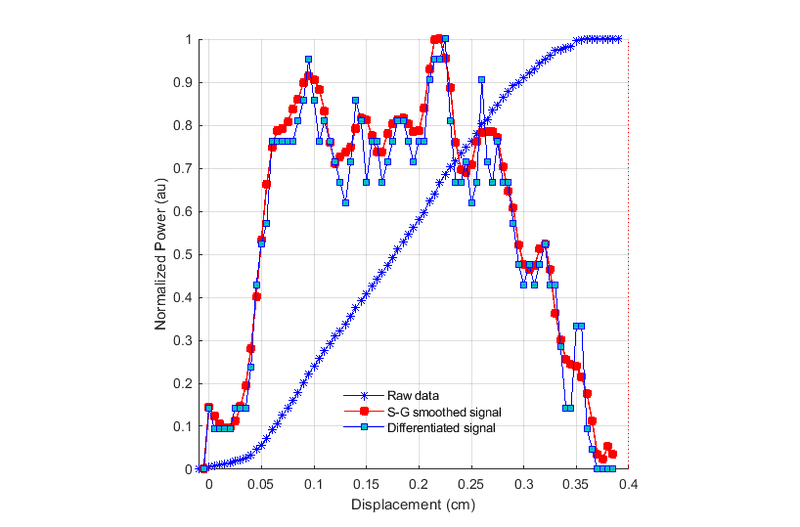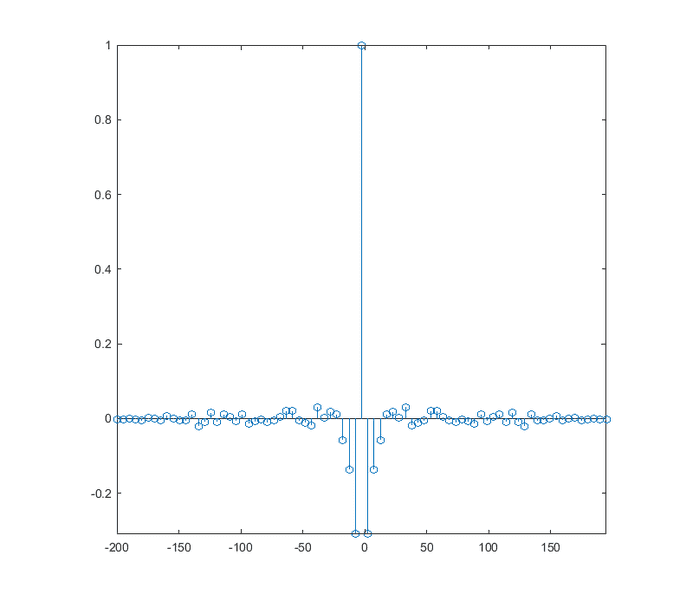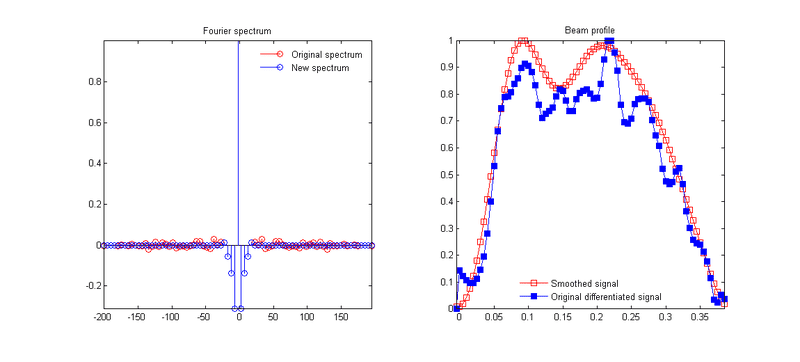# Fourier smoothing and Savitzky-Golay filtering

• I
roam
I am trying to recover a laser beam profile using numerical differentiation of the data obtained from a "knife-edge scan". I am trying to select between two different methods to smooth out the numerical noise.

Here is my raw data and the derivative:Here, I arbitrarily chose the 13 points, 4th order Savitzky-Golay method.

And here is the frequency spectrum of the derivative:I have read that high frequencies caused purely by noise can be identified and removed in Fourier space, while the noise contamination of the low order components cannot be eliminated. So, in my spectrum how do we tell where the noise band starts?

Due to instrumental limitations, I am restricted to 2 decimal places when making measurements. If we had data with more significant figures, would that make the noise band more discernable?

In general, how does the Fourier smoothing compare to the Savitzky-Golay method?

#### Attachments

Differentiation of a set of measurements is equivalent to a "high-pass" filter, you remove the basic information and are left with the noise. If you really want the derivative of a measurement data set, I suggest you do a curve fitting of some sort and and do a symbolic differentiation of the fitted curve.

In general, how does the Fourier smoothing compare to the Savitzky-Golay method?

There’s a nice “Lecture Notes” article by R.W. Schafer, “What Is a Savitzky-Golay Filter,” in IEEE Signal Processing Magazine, July 2011, that discusses this very topic!

roam
Differentiation of a set of measurements is equivalent to a "high-pass" filter, you remove the basic information and are left with the noise. If you really want the derivative of a measurement data set, I suggest you do a curve fitting of some sort and and do a symbolic differentiation of the fitted curve.

All academic papers that I have seen use numerical differentiation. I tried what you suggested in another thread dealing with a similar problem. It doesn't work, because a linear fit shows a very good r2 value — yet you can't obtain the laser beam profile by differentiating it. The derivative of a quadratic and a cubic also show no resemblance to the actual laser beam.

The laser beam I am working with is similar to the Fresnel pattern you would get from a rectangular source field. But it has aberrations due to imperfections in manufacturing and the optics that are involved. So we don't know the underlying function describing the beam.

When I look at the burn patterns of the laser, its general features agree with the results of the numerical differentiation. It's those smaller wiggles that I think are caused by noise. Some papers use a simple moving average on the derivative, which I think hides useful information by flatting out the curve. One paper uses Savitzky-Golay. I thought about using Fourier filtering, but how would you decide what coefficients to remove?

There’s a nice “Lecture Notes” article by R.W. Schafer, “What Is a Savitzky-Golay Filter,” in IEEE Signal Processing Magazine, July 2011, that discusses this very topic!

Could you please explain? I had a cursory look at the paper and it doesn't really compare Fourier filtering with S-G filtering.

Could you please explain? I had a cursory look at the paper and it doesn't really compare Fourier filtering with S-G filtering.
The paper discusses the frequency (Fourier) domain interpretation of the S-G filter (see Fig. 5).

roam
The paper discusses the frequency (Fourier) domain interpretation of the S-G filter (see Fig. 5).

But they are comparing to Parks-McClellan algorithm, not the method that I am asking about (which is simply to exclude some of the coefficients from the Fourier spectrum).

But they are comparing to Parks-McClellan algorithm, not the method that I am asking about (which is simply to exclude some of the coefficients from the Fourier spectrum).
The frequency response of the S-G filter shows you exactly what effect it has on the spectrum. You can compare that to any Fourier filter you like.

All academic papers that I have seen use numerical differentiation. I tried what you suggested in another thread dealing with a similar problem. It doesn't work, because a linear fit shows a very good r2 value — yet you can't obtain the laser beam profile by differentiating it. The derivative of a quadratic and a cubic also show no resemblance to the actual laser beam.
If you really have to do a numerical differentiation, try using $y_{n}'=\frac{y_{n+1}-y_{n-1}}{x_{n+1}-x_{n-1}}$ instead of $y_{n}'=\frac{y_{n+1}-y_{n}}{x_{n+1}-x_{n}}$. The former does a better job numerically.

roam
Hi Svein,

If you really have to do a numerical differentiation, try using $y_{n}'=\frac{y_{n+1}-y_{n-1}}{x_{n+1}-x_{n-1}}$ instead of $y_{n}'=\frac{y_{n+1}-y_{n}}{x_{n+1}-x_{n}}$. The former does a better job numerically.

Yes, I have already used the central difference approximation.

Do you think it is possible to distinguish noise from the actual deterministic part of the signal by looking at the Fourier spectrum?The spectrum of the derivative (calculated using CDA) is shown in my first post...

Do you think it is possible to distinguish noise from the actual deterministic part of the signal by looking at the Fourier spectrum?The spectrum of the derivative (calculated using CDA) is shown in my first post...
I have done this and it works - sort of. Try throwing away everything above the 5. harmonic and then transfer back.

•roam
roam
I have done this and it works - sort of. Try throwing away everything above the 5. harmonic and then transfer back.

Interesting. I tried that, this is what I got:Is there a reason you chose the 5th harmonic? Is there any way to tell if we are oversmoothing?

#### Attachments

•